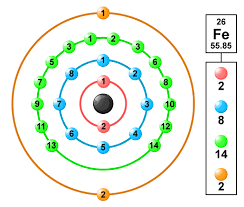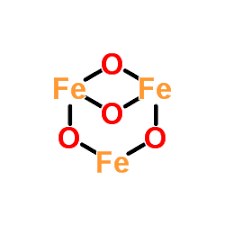# How Many Valence Electrons Are There in Iron?

Today we shall discuss the valency of another important element, i.e., What is Valency of Iron? As you know, valency is the measure of the capacity of a particular element to react and combine or displace the electrons of atoms of another element.

If the number of electrons in an atom is less than or equal to four, the number of valence electrons is equal to the number of electrons in its outer shell. If it is more than four, it is eight minus the number of electrons in the outer shell.

###Iron has an atomic number of 26. The valence electrons can vary from -2 to +6, but the most common valency it exhibits is +2 and +3.

### How Do You Find The Valency of IronIron (Fe) is a transitional metal that’s valency depends upon its oxidation state. When it combines with the sulfate radical to form ferrous sulfate, FeSO4, its valency must be +2, because the valency of the sulfate radical, as determined from the bond it forms with hydrogen, is -2.
In Iron the electronic configuration of Fe = 1s2 2s2 2p6 3s2 3p6 4s2 3d6

### What is the Valency of Iron in Fe3O4Oxide ions tend to have a 2- charge. The overall charge of Fe3O4 is 3(Fe) + 4(-2) = 0, which means that the valency of iron in Fe3O4 is +8/3. But since having fractional valencies is not possible (you can only lose a whole number of electrons, but not fractions of an electron), this is only an average valency for the iron atoms in Fe3O4.